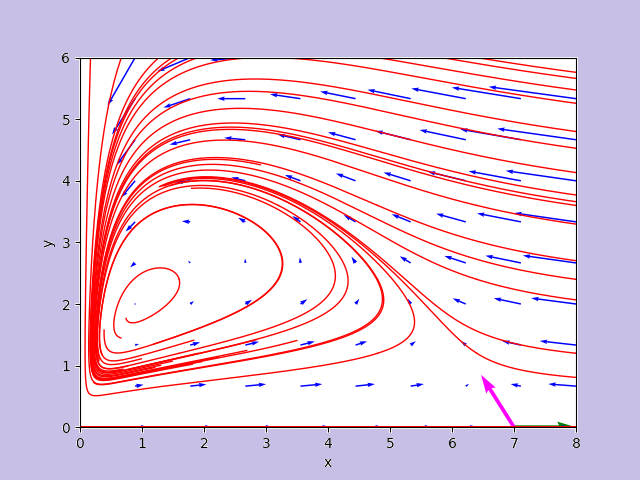## Sections

### Neural Networks

Studies, experiments and examples about deep learning machine models based on different topologies of neural networks: multilayer perceptrons, convolutional and recurrent layers, long-short-term-memory cells. Applications of neural networks to fit mathematical objects, to analyze texts, images, sounds and videos, to search for recurrent patterns in numerical series, to solve differencial equations. Code strictly original written in Python 3 with TensorFlow and/or PyTorch or in Julia with Flux, working and freely available on GitHub.
More...

### Quantum Computing

Studies, experiments and examples about programs written for quantum computers and correspondent simulators. Algorithms that uses combinations of quantum gates, qubit superposition, entanglement, measure collapse. Analysis of results got from the execution of programs on true quantum computers. Code strictly original written in most common languages for the quantum programming like QASM, Q# and Python con Qiskit, working and freely available on GitHub.
More...

## Highlights### Analyzer of a nonlinear autonomous dynamical system on the plane by Hartman-Grobman theorem

The post presents a Python program that analyzes the behavior of a nonlinear and autonomous dynamic system on the plane given two differential equations put into a system. Precisely, the program calculates the critical points, calculates the Jacobian at the critical points, verifies that they are hyperbolic and in this case it studies the behavior at the critical points linearizing around these points by applying Hartman-Grobman theorem. More...